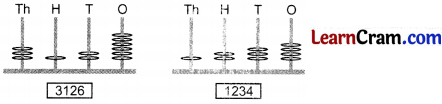# DAV Class 3 Maths Chapter 1 Worksheet 1 Solutions

The DAV Class 3 Maths Book Solutions and DAV Class 3 Maths Chapter 1 Worksheet 1 Solutions of Numbers upto 9999 offer comprehensive answers to textbook questions.

## DAV Class 3 Maths Ch 1 WS 1 Solutions

Question 1.
What number does the abacus show?

a.Solution:
3415

b.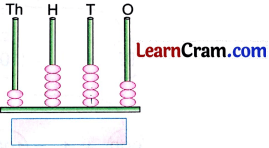Solution:
2553c.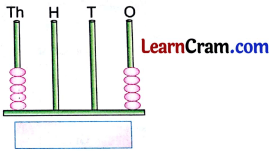Solution:
5005

d.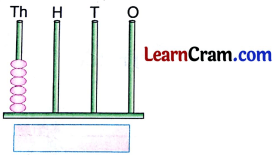Solution:
6000

e.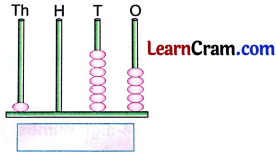Solution:
1075

f.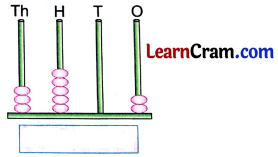Solution:
3502Question 2.
Represent the following numbers on the abacus.

(a)Solution:(b)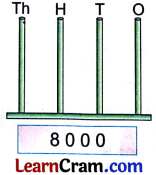Solution: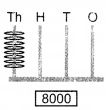(c)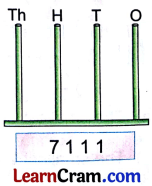Solution: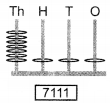### DAV Class 3 Maths Chapter 1 Worksheet 1 Notes

• 0, 1, 2, 3, 4, 5, 6, 7, 8 and 9 are digits used to write numbers.
• Numbers have one or more digits.
• Numbers like 0, 2, 4, 5 and 7 have just one digit. They are one-digit numbers.
• Numbers like 26, 32, 45, 85 and 92 are two-digit numbers.
• Numbers like 200, 302, 407 and 873 are some three-digit numbers.

Question 1.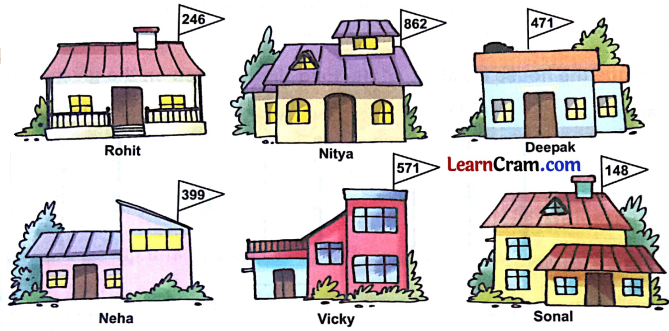House numbers of some children are given here. Write the number names of their house numbers in the space provided.Solution:

 Child Name House Number Number Name (a) Rohit 246 Two hundred forty six. (b) Nitya 862 Eight hundred sixty two. (c) Deepak 471 Four hundred seventy one. (d) Neha 399 Three hundred ninty nine. (e) Vicky 571 Five hundred seventy one (f) Sonal 148 One hundred forty-eightQuestion 2.
Write the house numbers of the following children and arrange them in ascending order.Solution:

 Child name Rohit Sonal Nitya Deepak Neha House No. 246 148 862 471 399

Ascending order: 148, 246, 399, 471, 862.

Question 3.
Arrange the house numbers of the following children on given abacus.Solution:
247.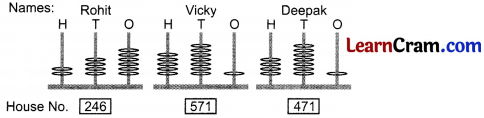Question 4.
What is one more than the house number of Rohit?
Solution:
247.

Question 5.
What is one less than the house number of Deepak?
Solution:
470.

Question 6.
How much more is the house number of Vicky than Deepak?
Solution:
100.

Question 7.
Find the sum of the house numbers of Sonal and Nitya.
Solution:
1010.

Question 8.
Write the name of the child whose house number is—

(a) the smallest.
Solution:
148 Sonal

(b) the greatest.
Solution:
862 Nitya

(c) greater than 500 but less than 800.
Solution:
571 Vicky

(d) between 350 and 45.
Solution:
399 NehaFour- Digit Numbers: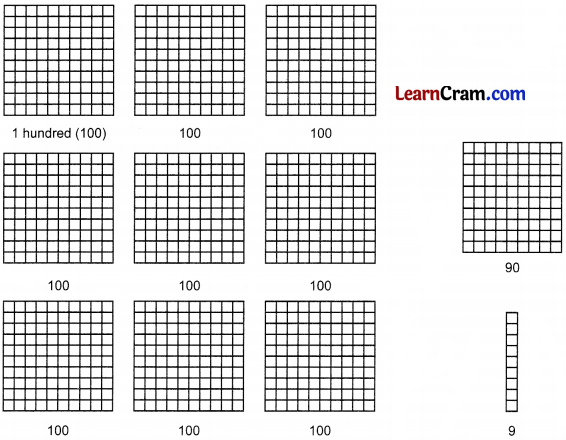999 + 1 = 1000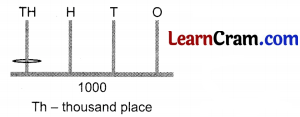• 999 is the greatest 3-digit number.
• 1000 is the smallest 4-digit number.
• 9999 is the greatest 4-digit number.Examples:
Some more 4-digit numbers on abacus.